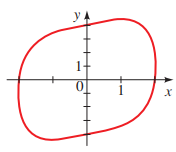# The x- and y- intercepts for the equation x 4 + y 2 − x y = 16 and the graph of the equation is provided below,### Precalculus: Mathematics for Calcu...

6th Edition
Stewart + 5 others
Publisher: Cengage Learning
ISBN: 9780840068071### Precalculus: Mathematics for Calcu...

6th Edition
Stewart + 5 others
Publisher: Cengage Learning
ISBN: 9780840068071

#### Solutions

Chapter 1.8, Problem 55E
To determine

## To calculate: The x- and y- intercepts for the equation x4+y2−xy=16 and the graph of the equation is provided below,Expert Solution

The x-intercepts are 2 and 2andy-intercepts are 4 and 4 .

### Explanation of Solution

Given information:

The equation x4+y2xy=16 and the graph of the equation is provided below,Formula used:

The x-intercepts are the points on x-axis where the graph of the equation intersects the x-axis.

The y-intercepts are the points on y-axis where the graph of the equation intersects the y-axis.

Calculation:

Consider the provided equation x4+y2xy=16 and the graph of the equation is sketched below,Observe from the graph that the curve intersects x-axis at two points 2 and 2 also y-axis at two points 4 and 4 .

Recall that the x-intercepts are the points on x-axis where the graph of the equation intersects the x-axis.

Substitute y=0 in the equation x4+y2xy=16 ,

x4+02x(0)=16x4=16x=±2

Therefore, x-intercepts are 2 and 2.

Recall that the y-intercepts are the points on x-axis where the graph of the equation intersects the y-axis.

Substitute x=0 in the equation x4+y2xy=16 ,

04+y2(0)y=16y2=16y=±4

Therefore, y-intercepts are 4 and 4 .

Thus, the x-intercepts are 2 and 2andy-intercepts are 4 and 4 .

### Have a homework question?

Subscribe to bartleby learn! Ask subject matter experts 30 homework questions each month. Plus, you’ll have access to millions of step-by-step textbook answers!